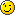# Labs 08

The tools we will use are

Princess is a theorem prover for Presburger arithmetic.

MONA is a tool that translates formulas into finite-state automata. It supports WS1S and WS2S.

## Princess

supports

• Presburger arithmetic
• uninterpreted predicates (tool may not terminate on these)
\existentialConstants {
int A, B;
}

\predicates {
divides(int, int);
}

\problem {
\forall int x; divides(x, x)
-> \forall int x, y; (divides(x, y) -> divides(x, y+x) & divides(x, y-x))
->
divides(A, 51) & A < 51 & A > 1 &
divides(B, 42) & divides(B, 49) & B > 1
}

This will produce a satisfying assignment, if one exists. To get the most general answer, use the option “+mostGeneralConstraint”. (Note that on the above the tool does not terminate.)

#### Exercises

##### 1)

Use Princess to solve the constraint system we had in class.##### 2)

One possible application of the tool is in code simplification. Use Princess to eliminate the temporary variables in the following code:

  t1 = a + b
t2 = c + d
t3 = t1 + e
sum = t3 + t2
##### 3)

Use Princess to solve this puzzle: If Frank and Sam total their ages, the answer is 49. Frank is twice as old as Sam was when Frank was as old as Sam is now. How old are Frank and Sam?

##### 4)

Consider the following problem: You have 4 weights, which have to be combined in the following way

w1 + 3 * w2 + 9 * w3 + 27 * w4 == weight

and the weights can be between -1 and 1.

• Does this problem have a solution for weight = 42?
• Use Princess to find a value for weight, for which this formula is satisfiable. (Without trying out all values between -100 and 100 if possible).
##### 5)

Use princess to solve the following optimization problem:## MONA syntax

MONA supports

• booleans, integers and sets of integers
• addition and subtraction, but only with a constant, i.e. x + 1 is allowed, but x + y or 1 + x is not.
• set operations (empty, union, inter, \, {…}, sub (), =, ~=)
• boolean operators (~, &, |, ⇒, ⇔)
• quantifiers(ex0, ex1, ex2, all0, all1, all2)
• <, >, ⇐, >=, =, ~=
• min{…}, max{…}
• only finite sets
• predicates
var2 P,Q;
P\Q = {0,4} union {1,2};

pred even(var1 p) =
ex2 Q: p in Q
& (all1 q:
(0 < q & q <= p) =>
(q in Q => q - 1 notin Q)
& (q notin Q => q - 1 in Q))
& 0 in Q;

var1 x;
var0 A;

A & even(x) & x notin P;

#### Exercises

##### 1)

Try out what MONA produces for the automata for,,and also what happens when you negate your formula.

##### 2)

Use MONA to show that.

#### Optional, for those who are fast

Derive the Hoare triple for this piece of code and show that it holds using Princess

var content : set;
var size : integer;
invariant I ⇐⇒ (size = |content|);
procedure insert(e : element)
maintains I
requires |e| = 1 ∧ |e ∩ content| = 0
ensures size′> 0
{
content := content ∪ e;
size := size + 1;
}